# Cumulative Portfolio Credit Loss

## Definition

At each one of the future timepoints k we will experience credit losses captured by the random variables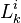$L^i_k$.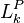$L^{P}_{k}$ is the observed value of the cumulated portfolio credit loss at intermediate times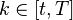$k \in [t, T]$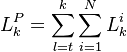$L^{P}_{k} = \sum_{l=t}^{k} \sum_{i=1}^{N} L^i_k$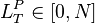$L^{P}_T \in [0, N]$ is the total portfolio credit loss variable (random and unknown at t), but fully realized and known at time T.